Technical Information Site of Power Supply Design

• Basic Knowledge
• SiC Power Device
• SiC MOSFETs: Behavior of Gate-Source Voltage in a Bridge Configuration
• Behavior of the Gate-Source Voltage During Low-side Switch Turn-on

2021.02.10 SiC Power Device

# Behavior of the Gate-Source Voltage During Low-side Switch Turn-on

SiC MOSFETs: Behavior of Gate-Source Voltage in a Bridge Configuration

The previous article briefly explained the currents and voltages occurring due to changes in VDS and ID during switching operation of the gate driving circuit in a SiC MOSFET bridge configuration. This article explains in detail the behavior upon LS turn-on.

Behavior of the Gate-Source Voltage During Low-side Switch Turn-on

When LS turns on, first ID changes (see the schematic waveform diagram T1 below). At this time the LS ID is increasing and the HS ID is decreasing, and so due to the events (I) shown in the equivalent circuit diagram below, the emf of equation (1) appears with the indicated polarity. This equation (1) is the same as that presented in the previous article. The current due to this emf charges CGS with the source side as positive, and so while VGS is forced downward on LS, VGS on HS is pulled to the negative side, and a negative surge is caused (in the interval T1 in the schematic waveform diagram for VGS).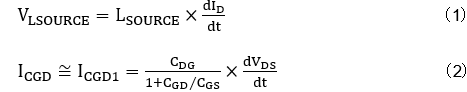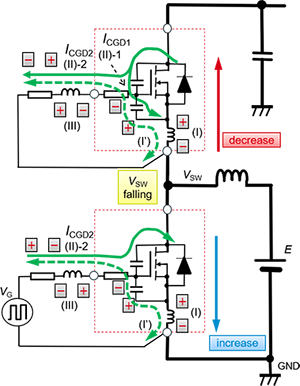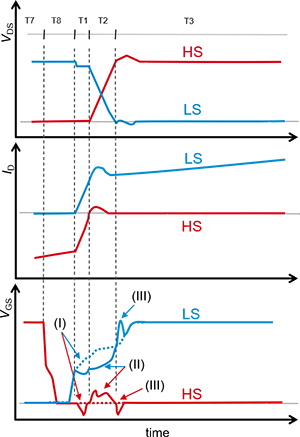When the change in ID ends, the LS VDS falls (time interval T2 in the schematic waveform diagram). Hence the currents given by equation (2) flow as indicated by (II)-1 and (II)-2 in the equivalent circuit diagram, and voltage rises expressed by equations (3) and (4) respectively occur in VGS.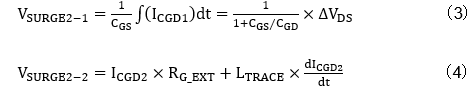Immediately after VDS begins changing, the main rise in VGS is due to the component of equation (3), but as time passes the rise in VGS due to equation (4) also begins. That is, the CGD/CGS ratio of the MOSFET, RG_EXT in the driving circuit, and the inductance LTRACE of the gate driving signal trace, all have large effects.

As indicated in the equivalent circuit diagram, the HS (II-2) current ICGD2 flows in the direction that raises VGS. Hence HS, which normally should be turned off, gradually begins turn-on operation as VGS is raised. This is called self turn-on. When HS self turn-on occurs, it overlaps with LS turn-on operation so that the HS and LS MOSFETs are turned on simultaneously, and a through-current flows.

ICGD2 continues to flow until the LS turn-on operation is completed and is accumulated in LTRACE, but when the change in VSW is completed, the current is annihilated and LTRACE generates a voltage. This is the events (III). Depending on the switching conditions such as the value of RG_EXT, ICGD2 can be as large as several amperes, resulting in a substantial emf.

The gate-source voltage when LS is turned on exhibits the behavior seen in the schematic waveform diagram due to the above-described events (I), (II), and (III). The numbers in the schematic waveform diagram and the equivalent circuit diagram represent the same events. The broken-line waveform for VGS represents the ideal waveform.

Effect of External Gate Resistors

Below we present double-pulse test results when LS is turned on in a SiC MOSFET bridge configuration. Here the waveforms in (a) are for the case in which the external gate resistors RG_EXT are 0 Ω, and (b) is for the case of 10 Ω. In the diagram, (I), (II), and (III) refer to the above-described events.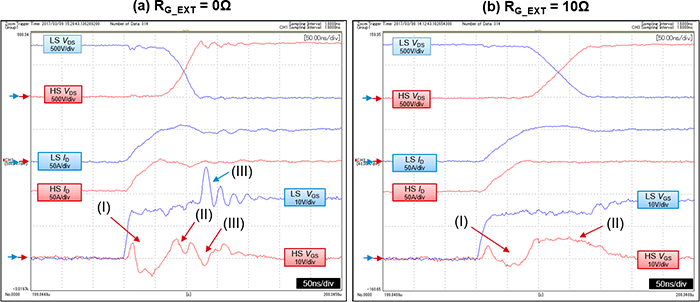From comparison of the waveforms in (a) and (b), it is seen that for smaller values of RG_EXT, the decrease in VGS due to the event (I) is larger. Moreover, because switching speeds are extremely fast, the event (III) occurs prominently in (a), but because RG_EXT is 0 Ω, almost no waveform for event (II) is observed. However, in (b) the raising of VGS by the event (II)-2 and RG_EXT appears prominently.

As is clear from these results as well, in order to reduce the rise in VGS due to the event (II)-2 that induces self turn-on of HS while LS is turned on, the value of the external gate resistor RG_EXT during HS turn-off must be lowered. However, in general RG_EXT is made the same for both HS and LS, and consequently when RG_EXT is lowered the LS dVDS/dt increases, and as equation (1) indicates, the HS ICGD increases. As a result, an increase in the HS surge is caused, as is seen from equation (4).

One method of countering this is to separately set RG_EXT at turn-on and at turn-off, reducing the value of RG_EXT only at turn-off. As a widely used method, a diode may be employed, as in the diagram on the right. In this method, the resistor that operates during turn-on is RG_ON alone, but during turn-off the diode is conducting, and the resistance is that of the parallel resistors RG_ON and RG_OFF. Hence the resistance value during turn-off is lower than that during turn-on.In contrast with the schematic waveform diagram initially explained, the HS VGS waveform swings briefly to the positive side immediately before event (I) because the emf due to LSOURCE in the instant that the current of event (I) begins to flow passes through CGS and is observed immediately thereafter.

The next article will explain in detail the behavior when LS turns off.

#### Key Points:

・In a bridge configuration, due to MOSFET gate capacitances and changes in VDS and ID resulting from switching, in some cases HS self turn-on may occur when the LS switch turns on.

・One method to deal with self turn-on involves reducing the values of external gate resistors, but in order to avoid effects on other operation, measures are necessary to reduce the gate resistance only during HS turn-off.